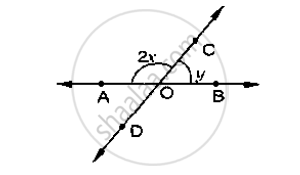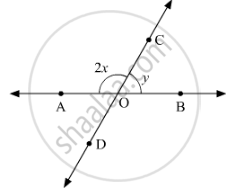# In the Below Fig, Rays Ab and Cd Intersect at O. Determine Y When X = 60° - Mathematics

In the below fig, rays AB and CD intersect at O.
Determine y when x = 60°#### Solution 1

Given x = 60°

y = ?

∠AOC, ∠BOC are linear pair of angles

∠AOC + ∠BOC = 180°

⇒ 2x + y = 180°

⇒  2 × 60 + y = 180°          [ ∵x = 60°]

⇒  y = 180° -120°

⇒ y = 60°

#### Solution 2

Rays ABand CD intersect at point O.Therefore, ∠AOCand ∠BOCform a linear pair.

Thus,

∠AOC +∠BOC = 180°

2x +y =180°       (1)

On substituting x = 60° :

2x + y = 180°

2(60°) + y = 180°

120° + y= 180°

y = 180°- 120°

y = 60°

Concept: Concept of Parallel Lines
Is there an error in this question or solution?

#### APPEARS IN

RD Sharma Mathematics for Class 9
Chapter 10 Lines and Angles
Exercise 10.3 | Q 6.1 | Page 23